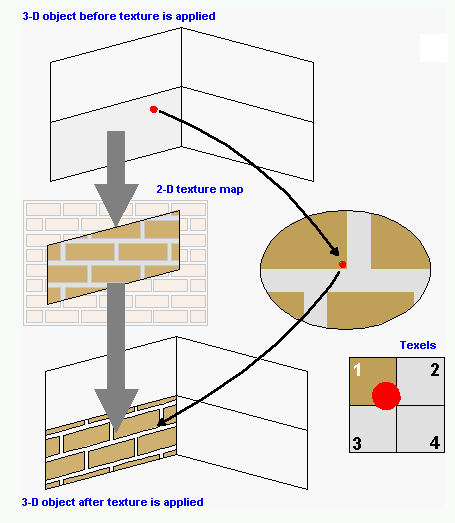# bilinear interpolation

Also found in: Wikipedia.

## bilinear interpolation

A texture mapping technique that produces a reasonably realistic image, also known as "bilinear filtering" and "bilinear texture mapping." An algorithm is used to map a screen pixel location to a corresponding point on the texture map. A weighted average of the attributes (color, alpha, etc.) of the four surrounding texels is computed and applied to the screen pixel. This process is repeated for each pixel forming the object being textured.

The term bilinear refers to the performing of interpolations in two dimensions (horizontal and vertical). The top and bottom pairs of each texel quadrant are averaged (horizontal) and then their results are averaged (vertical). This method is often used in conjunction with MIP mapping. See texture map, MIP mapping, point sampling and trilinear interpolation.

Bilinear MappingEach screen pixel of the object is mapped onto the corresponding texel in the texture map. The red dots are an example of one pixel. The attributes of the weighted average of the four nearest texels is applied to the screen pixel. Two horizontal interpolations are made (texels 1-2 and 3-4), and then the results are averaged together for the vertical interpolation. (Redrawn from illustration courtesy of Intergraph Computer Systems.)
Copyright © 1981-2019 by The Computer Language Company Inc. All Rights reserved. THIS DEFINITION IS FOR PERSONAL USE ONLY. All other reproduction is strictly prohibited without permission from the publisher.
Mentioned in ?
References in periodicals archive ?
(iv) Bilinear is bilinear interpolation, when the point value in the new image is calculated by linear interpolation between the values of the four nearest points;
The triangle filter, also known as the bilinear interpolation filter, gives a smooth, natural gradient pass between pixels when upsampling, in contrast to the box filter (Thyssen, 2017).
 proposed a reversible data hiding method based on DE and bilinear interpolation. In their scheme, two secret bits could be embedded into one cover pixel.
Regarding the blurred image [I.sub.s], it was generated by downsampling and upsampling the denoised LR image [??] with bilinear interpolation.
Nearest neighbor interpolation, bilinear interpolation, and cubic convolution interpolation are widely used [13, 14].
The classical interpolation methods include nearest interpolation, bilinear interpolation, and bicubic interpolation .
Figure 1 shows an example of upscaling (s = 3/2) with bilinear interpolation in the ith row of image B.
Exposure buildup factor ([beta]) was computed by (3)-(5) according to bilogarithmic interpolation (BI) modified from bilinear interpolation as seen in Figure 2(b) [14,15].
Specifically, the Coons patch is obtained as follows: (1) consider the sum of the two linear interpolations between the two sets of opposite boundary lines; (2) from this subtract a bilinear interpolation through the four corner points.
The intensity value of the point was assigned by the bilinear interpolation of the four enclosing pixels on the scan.
Another complicated methodology is named bilinear interpolation 
On the reference element, denote the bilinear interpolation operator [mathematical expression not reproducible] by

Site: Follow: Share:
Open / Close